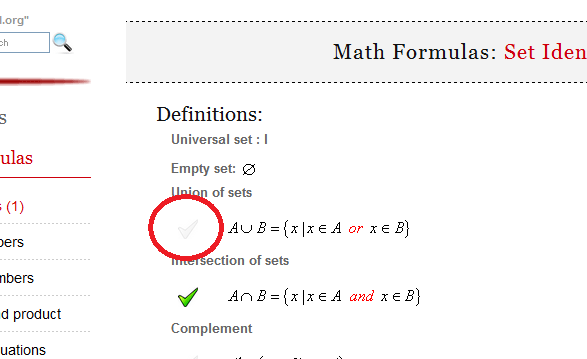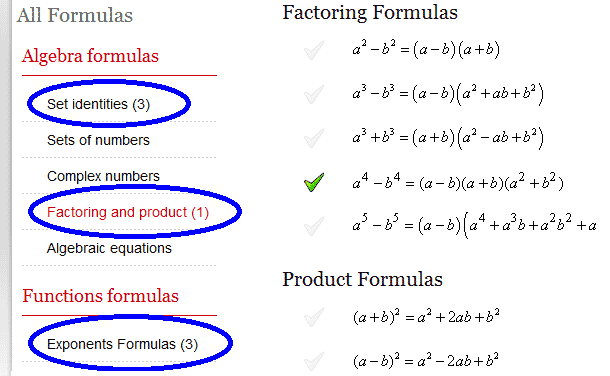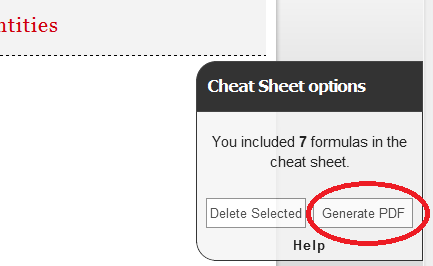Math Calculators, Lessons and Formulas

It is time to solve your math problem

mathportal.org

You included 0 formulas in your custom cheat sheet.

Cheat Sheet options

## How to generate cheat sheet ?

To create cheat sheet first you need to select formulas which you want to include in it. To select formula click at picture next to formula.You can choose formulas from different pages.After you have selected all the formulas which you would like to include in cheat sheet, click the "Generate PDF" button.# Math Formulas: Lines in three dimensions

### Line forms

Point direction form:

 $$\frac{x-x_1}{a} = \frac{y - y_1}{b} = \frac{z-z_1}{c}$$

Two point form:

 $$\frac{x-x_1}{x_2-x_1} = \frac{y - y_1}{y_2-y_1} = \frac{z-z_1}{z_2-z_1}$$

Parametric form:

 \begin{aligned} x &= x_1 +t\,\cos \alpha \\ y &= y_1 +t\,\cos \beta \\ z &= z_1 +t\,\cos \gamma \end{aligned}

### Distance between two lines in 3 dimensions

The distance from $P_2(x_2,y_2,z_2)$ to the line through $P_1(x_1,y_1,z_1)$ in the direction $(a,b,c)$ is

 $$d = \sqrt{ \frac{\left[c(y_2-y_1)-b(z_2-z_1)\right]^2 + \left[a(z_2-z_1)-c(x_2-x_1)\right]^2 + \left[b(x_2-x_1)-a(y_2-y_1)\right]^2} {a^2 + b^2 + c^2 } }$$

The distance between two lines. First one through $P_1(x_1,y_1,z_1)$ in direction $(a_1,b_1,c_1)$, Second one: through $P_2(x_2,y_2,z_2)$ in direction $(a_2,b_2,c_2)$ is:

 $$d = \frac{ \begin{vmatrix} x_2-x_1 & y_2-y_1 & z_2-z_1 \\ a_1 & b_1 & c_1 \\ a_2 & b_2 & c_2 \end{vmatrix} } { \sqrt{\begin{vmatrix} b_1 & c_1 \\ b_2 & c_2 \end{vmatrix}^2 + \begin{vmatrix} c_1 & a_1 \\ c_2 & a_2 \end{vmatrix}^2 + \begin{vmatrix} a_1 & b_1 \\ a_2 & b_2 \end{vmatrix}^2 }}$$

The two lines intersect if:

 $$\begin{vmatrix} x_2-x_1 & y_2 - y_1 & z_2 - z_1 \\ a_1 & b_1 & c_1 \\ a_2 & b_2 & c_2 \end{vmatrix} = 0$$